Home > CC4 > Chapter 11 > Lesson 11.1.3 > Problem11-36

11-36.
1. Solve the equations below. Explain your steps for parts (a) and (c). Check your solutions, if possible. Homework Help ✎

2.  a.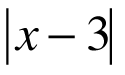+ 3 = 4 b. (x − 5)3 = 8 c.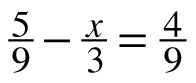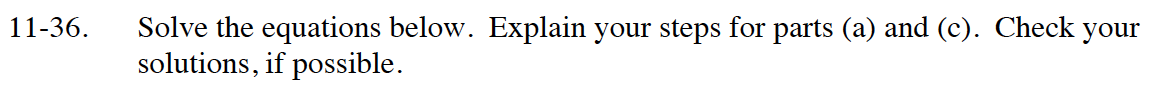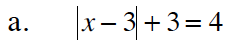First, isolate the absolute value term.

The value inside the absolute value signs is equal to 1 or −1. Write two equations and solve each of them for x.
x − 3 = 1 x − 3 = −1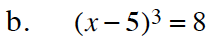What is the cube root of 8?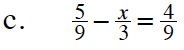If you multiply each term by the common denominator, 9, and use Giant Ones, you will eliminate the denominators.